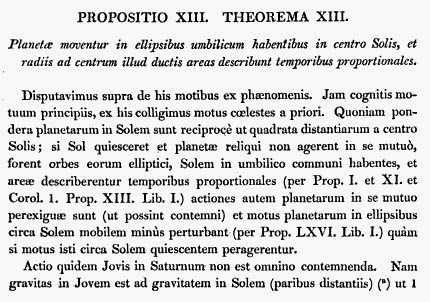### De mundi systemate

by @ulaulaman about #IsaacNewton #physics #gravity
I published this post some years ago (archived version), but for unilateral decision of the online publisher, it is deleted, so I decide to recover it.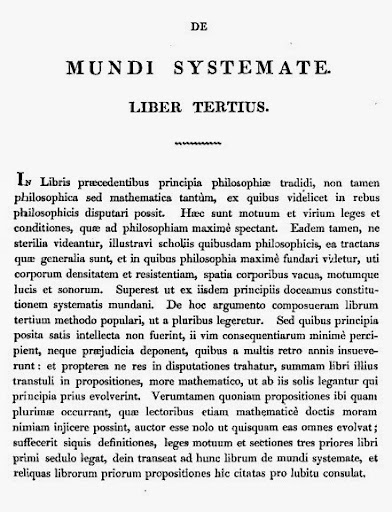It's the third pard o Newton's Philosophiae Naturalis Principia Mathematica, one of the most famous physics tractatus (latin world to treatise). In 2010 Dave Richeson proposes An amazing paragraph from Euler's Introductio, a wonderful post in which he extraxts some Euler's quotes from Introductio in analysin infinitorum (Introduction to analysis of the infinite). So, because I'm working on a learning project about gravity, I decide to propose an analouge post about De mundi systemate (On worlds' system). For english version of the following Newton's quotes I'll ask help to Andrew Motte.
We began with Propositio II (Proposition II):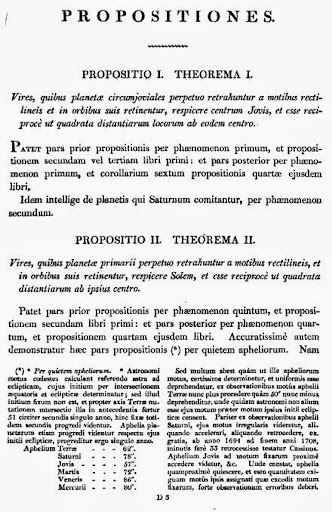Forces, by which the primary planets are continually drawn off from rectilinea motion, and retained in their orbits, come from the Sun, and they are reciprocally as the square of the distances from the centre.
In mathematical world, it means: $F = \frac{K}{r^2}$ Similarly the interaction between Moon and Earth: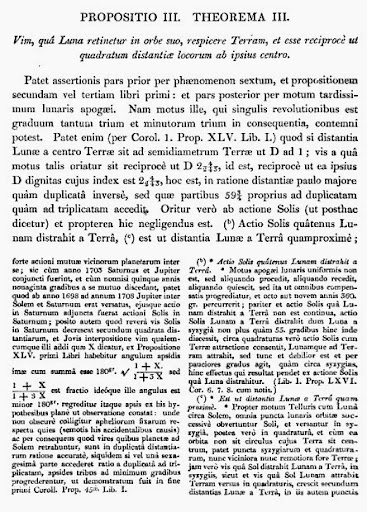Force, by which the Moon is retained in its orbit, tends to the Earth, and are reciprocally as the square of the distances by centre.
The following quote (Proposition VII) is very interesting: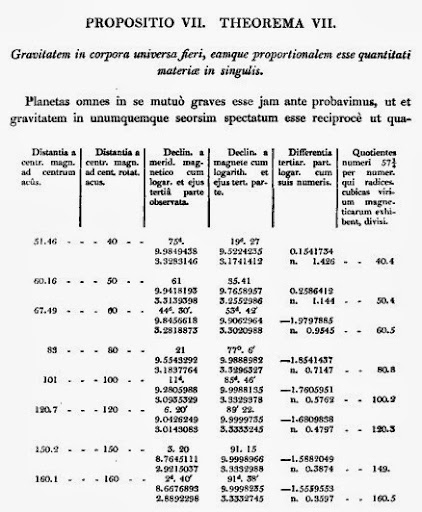The weight is in all bodies, and it's proportional to matter which they contain
In this quote we can see the first use of gravity, but I think that Newton means the translation that I proposed.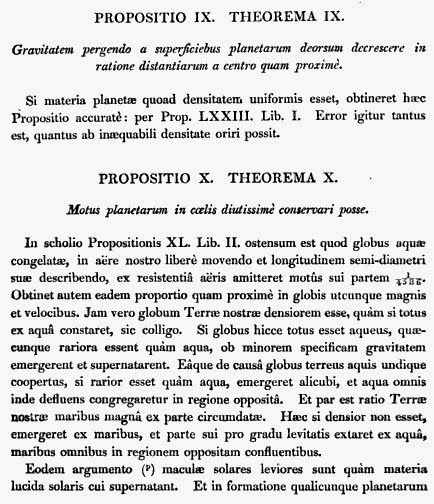That it means that gravity under planete's surface decreases proportionally to the distance from the centre: this is the classic problem of a tube through the earth passing to the centre. In order to obtain Newton's result we can think like the great mathematician: we consider the relation between mass, volume and density. Using modern mathematics we can write: $M = \rho V = k r^3$ Using it in the following expression: $a= \frac{GM}{r^2}$ we obtain $a=kr$ Proposition XIII consists in first and second Kepler's law: